×#### Thank you for registering.

One of our academic counsellors will contact you within 1 working day.

Click to Chat

1800-1023-196

+91-120-4616500

CART 0

• 0

MY CART (5)

Use Coupon: CART20 and get 20% off on all online Study Material

ITEM
DETAILS
MRP
DISCOUNT
FINAL PRICE
Total Price: Rs.

There are no items in this cart.
Continue Shopping• Complete JEE Main/Advanced Course and Test Series
• OFFERED PRICE: Rs. 15,900
• View Details

```Chapter 9: Triangle and its Angles Exercise – 9.2

Question: 1

The exterior angles, obtained on producing the base of a triangle both ways are 104° and 136°. Find all the angles of the triangle.

Solution:∠ACD = ∠ABC + ∠BAC [Exterior angle property]

Now ∠ABC = 180° − 136° = 44° [Linera pair]

∠ACB = 180° − 104° = 76° [Linera pair]

Now, In ΔABC

∠A + ∠ABC + ∠ACB = 180° [Sum of all angles of a triangle]

⇒ ∠A + 44° + 76° = 180°

⇒ ∠A = 180° − 44° − 76°

⇒ ∠A = 60°

Question: 2

In a triangle ABC, the internal bisectors of ∠B and ∠C meet at P and the external bisectors of ∠B and ∠C meet at Q. Prove that ∠BPC + ∠BQC = 180°.

Solution:

Let ∠ABD = 2x and ∠ACE = 2y∠ABC = 180° − 2x [Linera pair]

∠ACB = 180° − 2y [Linera pair]

∠A + ∠ABC + ∠ACB = 180° [Sum of all angles of a triangle]

⇒ ∠A + 180° − 2x + 180° − 2y = 180°

⇒ −∠A + 2x + 2y = 180°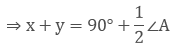Now in ΔBQC

x + y + ∠BQC = 180° [Sum of all angles of a triangle]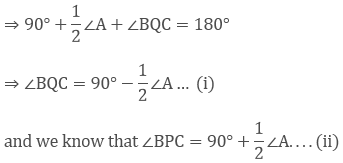Adding (i) and (ii) we get ∠BPC + ∠BQC = 180°

Hence proved.

Question: 3

In figure 9.30, the sides BC, CA and AB of a triangle ABC have been produced to D, E and F respectively. If ∠ACD = 105° and ∠EAF = 45°, find all the angles of the triangle ABC.

Solution:∠BAC = ∠EAF = 45° [Vertically opposite angles]

∠ABC = 105° − 45° = 60° [Exterior angle property]

∠ACD = 180° − 105° = 75° [Linear pair]

Question: 4

Compute the value of x in each of the following figures: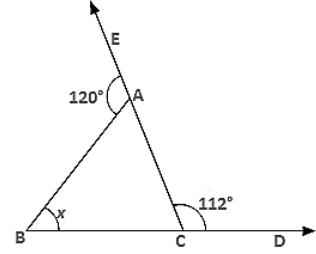(i)
(ii)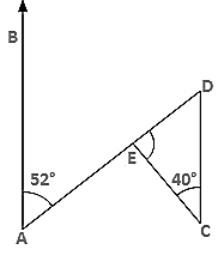(ii)
(iv)

Solution:

(i)  ∠BAC = 180° − 120° = 60° [Linear pair]

∠ACB = 180° − 112° = 68° [Linear pair]

∴ x = 180° − ∠BAC − ∠ACB

= 180° − 60° − 68° = 52° [Sum of all angles of a triangle]

(ii) ∠ABC = 180° − 120° = 60° [Linear pair]

∠ACB = 180° − 110° = 70° [Linear pair]

∴ e∠BAC = x

= 180° − ∠ABC − ∠ACB = 180° − 60° − 70° = 50° [Sum of all angles of a triangle]

(iii) ∠BAE = ∠EDC = 52° [Alternate angles]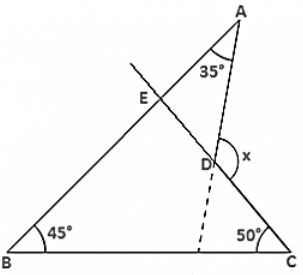∴ ∠DEC = x = 180° − 40° − ∠EDC

= 180° − 40° − 52°

= 180° − 92° = 88° [Sum of all angles of a triangle]

(iv) CD is produced to meet AB at E.

∠BEC = 180° − 45° − 50° = 85° [Sum of all angles of a triangle]

∠AEC = 180° − 85° = 95° [Linear pair]

∴ x = 95° + 35° = 130° [Exterior angle property].

Question: 5

In figure 9.35, AB divides ∠DAC in the ratio 1: 3 and AB = DB. Determine the value of x.Solution:

Let ∠BAD = Z, ∠BAC = 3Z

⇒ ∠BDA = ∠BAD = Z (∵ AB = DB)

Now ∠BAD + ∠BAC + 108° = 180° [Linear pair]

⇒ Z + 3Z + 108° =180°

⇒ 4Z = 72°

⇒ Z = 18°

∠ADC + ∠ACD = 108° [Exterior angle property]

⇒ x + 18° = 180°

⇒ x = 90°

Question: 6

ABC is a triangle. The bisector of the exterior angle at B and the bisector of ∠C intersect each other at D. Prove that ∠D = 1/2∠A.

Solution:

Let ∠ABE = 2x and ∠ACB = 2y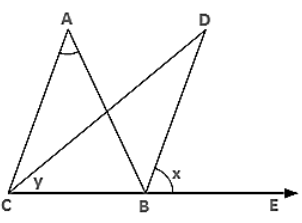∠ABC = 180° − 2x [Linear pair]

∴ ∠A = 180° − ∠ABC − ∠ACB [Angle sum property]

= 180° − 180° + 2x + 2y

= 2(x − y) ..... (i)

Now, ∠D = 180° − ∠DBC − ∠DCB

⇒ ∠D = 180° − (x + 180° − 2x) − y

⇒ ∠D = 180° − x − 180° + 2x − y

= (x − y)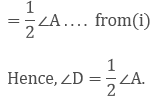Question: 7

In figure 9.36, AC ⊥ CE and ∠A:∠B: ∠C = 3: 2: 1, find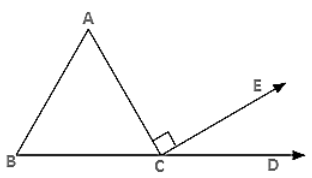Solution:

∠A: ∠B: ∠C = 3: 2: 1

Let the angles be 3x, 2x and x

⇒ 3x + 2x + x = 180° [Angle sum property]

⇒ 6x = 180°

⇒ x = 30° = ∠ACB

∴ ∠ECD = 180° − ∠ACB − 90° [Linear pair]

= 180° − 30° − 90°

= 60°

∴ ∠ECD = 60°

Question: 8

In figure 9.37, AM ⊥ BC and AN is the bisector of ∠A. If ∠B = 65° and ∠C = 33°, find ∠MAN.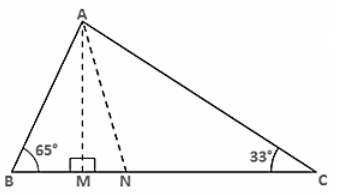Solution:

Let ∠BAN = ∠NAC = x [∵ AN bisects ∠A]

∴ ∠ANM = x + 33° [Exterior angle property]

In ΔAMB

∠BAM = 90° − 65° = 25° [Exterior angle property]

∴ ∠MAN = ∠BAN − ∠BAM = (x − 25)°

Now in ΔMAN,

(x - 25)° + (x + 33)° + 90° = 180° [Angle sum property]

⇒ 2x + 8° = 90°

⇒ 2x = 82°

⇒ x = 41°

∴ MAN = x − 25°

= 41° − 25°

= 16°

Question: 9

Solution: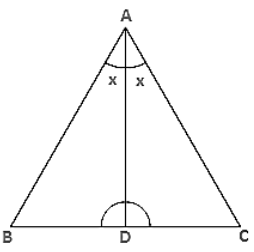∵ ∠C > ∠B                          [Given]

⇒ ∠C + x > ∠B + x              [Adding x on both sides]

Hence proved.

Question: 10

In triangle ABC, BD ⊥ AC and CE ⊥ AB. If BD and CE intersect at O, prove that ∠BOC = 180° - ∠A.

Solution:∠A + ∠AEO + ∠EOD + ∠ADO = 360°

⇒ ∠A + 90° + 90° + ∠EOD = 360°

⇒ ∠A + ∠BOC = 180° [∵ ∠EOD = ∠BOC vertically opposite angles]

⇒ ∠BOC = 180° − ∠A

Question: 11

In figure 9.38, AE bisects ∠CAD and ∠B = ∠C. Prove that AE ∥ BC.Solution:

Let ∠B = ∠C = x

Then,

∠CAD = ∠B + ∠C = 2x (exterior angle)

⇒ ∠EAC = x

⇒ ∠EAC = ∠C

These are alternate interior angles for the lines AE and BC

∴ AE ∥ BC

Question: 12

In figure 9.39, AB ∥ DE. Find ∠ACD.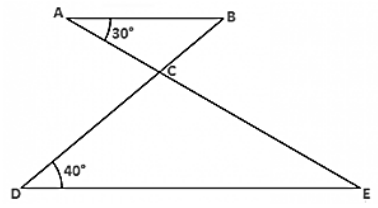Solution:

Since AB ∥ DE

∴ ∠ABC = ∠CDE = 40° [Alternate angles]

∴ ∠ACB = 180° − ∠ABC − ∠BAC

=180° − 40° − 30°

= 110°

∴ ∠ACD = 180° − 110° [Linear pair]

= 70°

Question: 13

Which of the following statements are true (T) and which are false (F):

(i) Sum of the three angles of a triangle is 180°.

(ii) A triangle can have two right angles.

(iii) All the angles of a triangle can be less than 60°.

(iv) All the angles of a triangle can be greater than 60°.

(v) All the angles of a triangle can be equal to 60°.

(vi) A triangle can have two obtuse angles.

(vii) A triangle can have at most one obtuse angles.

(viii) If one angle of a triangle is obtuse, then it cannot be a right angled triangle.

(ix) An exterior angle of a triangle is less than either of its interior opposite angles.

(x) An exterior angle of a triangle is equal to the sum of the two interior opposite angles.

(xi) An exterior angle of a triangle is greater than the opposite interior angles.

Solution:

(i) T

(ii) F

(iii) F

(iv) F

(v) T

(vi) F

(vii) T

(viii) T

(ix) F

(x) T

(xi) T

Question: 14

Fill in the blanks to make the following statements true:

(i) Sum of the angles of a triangle is _______ .

(ii) An exterior angle of a triangle is equal to the two ________ opposite angles.

(iii) An exterior angle of a triangle is always ________ than either of the interior opposite angles.

(iv) A triangle cannot have more than _______ right angles.

(v) A triangles cannot have more than _______ obtuse angles.

Solution:

(i) 180°

(ii) Interior

(iii) Greater

(iv) One

(v) One
```### Course Features

• 728 Video Lectures
• Revision Notes
• Previous Year Papers
• Mind Map
• Study Planner
• NCERT Solutions
• Discussion Forum
• Test paper with Video Solution